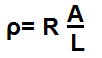﻿ Resistivity Calculator ﻿# Resistivity CalculatorResistance: Ω KΩ MΩ Length: m Area: m2
0.00Ω∙m

The resistivity calculator calculates the resistivity of a component based on its resistance value, its length, and its cross-sectional area. according to the above formula.

A user enters the resistance, R, the cross-sectional area, A, and the length of the component, L, and the result will automatically be calculated and shown. The resistivity result which is displayed above is in unit ohms∙meter (Ω∙m).

The resistivity is a measurement that calculates the amount of resistance a material will offer based on its intrinsic properities. The resistivity of a material is directly affected by the cross-secitional area of the material. The greater the cross-sectional area of a given substance, the greater the resistivity will be. Conversely, the smaller the cross-sectional area, the less the resistivity will be. Besides area, the resistivity is directly affected in the same way by the resistance of the material. The resistivity is inversely proportional to the length of the material. The greater the length of the material, the lower the resistivity. The smaller the length of the material, the greater the resistivity.

Thus a material with low resistivity will easily conduct electricity; it's a conductor. And a material with high resistivity will conduct electricity very poorly; it's an insulator.

The inverse of resistivity is conductivity, the ability of a material to conduct electricity. So if you take the result of this calculator and invert it by 1, it yields the conductivity result. Conductivity, σ= 1/ρ.

Related Resources

﻿